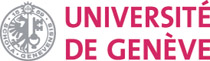Martin J. Gander Section de Mathématiques Rue du Conseil-Général 7-9 Case Postale 64 1205 Genève, Suisse martin.gander(at)unige.ch   Fax: +41 22 379 11 76Home Research Teaching Consulting

 Seminars Genève: - Analyse numérique McGill: - CSE - Applied Mathematics Conference Ascona 2013

 CSE at McGill New degree program in Computational Science and Engineering.

 :: Teaching Interests My teaching interests are both in Mathematics and Computer Science: in addition to undergraduate courses in both areas, I am interested and qualified to teach at the graduate level Scientific Computing, Numerical Differential Equations, Matrix Computations, Differential Equations, Parallel Computing, Numerical Dynamical Systems, Algorithms and Data Structures and Object Oriented Programing.

 :: Courses I teach this year in Geneva Analyse numérique des équations aux dérivées partielles: Beaucoup de phénomènes physiques peuvent être modélisés par des équations aux dérivées partielles, par exemple le flux d'un fluide dans un tuyau, les variations de température à l'intérieur d'un appartement ou la cuisson dans un four à micro-ondes. Mais la résolution de ces équations est souvent difficile et les méthodes analytiques suffisent rarement pour obtenir les résultats désirés. Ce cours est une introduction aux méthodes numériques modernes pour la résolution des équations aux dérivées partielles. Nous utiliserons Matlab pour développer des codes modèles simples, et Maple pour nous assister dans les calculs symboliques.

 :: Teaching Experience Differential Equations (McGill 2004): Introduction to differential equations: first order and linear second order equations, higher order equations, solution by series, Laplace transform and simple numerical methods. Ordinary Differential Equations (McGill, 2003): Introduction to ordinary differential equations: first and second order equations, linear equations, series solutions, Frobenius method, Laplace transforms and applications. Introduction à l'analyse numérique I (Genève, 2003): A first undergraduate course introducing students from mathematics and computer science to numerical integration, interpolation and approximations, numerical ordinary differential equations and linear systems. (40 students, 2 hours lecture, 1 hour exercises and 2 hours Fortran exercises) Introduction à l'analyse numérique II (Genève 2003): A second undergraduate course introducing students from mathematics and computer science to more advances topics in numerical analysis: iterative methods, eigenvalue and eigenvector computations and nonlinear systems. (40 students, 2 hours lecture, 1 hour exercises and 2 hours Fortran exercises) Numerical Differential Equations (McGill, 2000-2002): A graduate course in numerical methods for ordinary and partial differential equations, including Runge-Kutta, Linear Multistep and adaptive methods, finite elements, finite differences, finite volumes and spectral methods, fast solvers. (20 students, 4 hours lecture, exercises with Matlab and Maple) Numerical Analysis (McGill, 1999-2001): A graduate course in numerical methods, with linear and nonlinear systems, iterative methods, eigencomputations, quadrature, approximation. (20 students, 4 hours lecture, exercises with Matlab and Maple) Parallel Computing using Domain Decomposition (Summer course TU Denmark 1999): An introduction to domain decomposition methods. (25 students, 1 week lectures with exercises) Introduction to Scientific Computing (Stanford, 1996-1997): An advanced undergraduate, early graduate course in scientific computing using Maple and Matlab. (30 students, 3 hours lecture and exercises) Programming Paradigms (Solothurn, 1993): An introduction to the different programming paradigms using Object Pascal, Lisp and Prolog. (20 students, 2 hours lecture with exercises) Introduction to Computer Science II (Solothurn, 1990-1992): Algorithms and datastructures in Pascal. (18 students, 2 hours lecture with exercises) Introduction to Computer Science I (Solothurn, 1990-1992): An introduction to basic algorithms and programming in Pascal. (20 students, 2 hours lecture with exercises)

 :: Fun Problems and Talks A survey talk I gave at the university of Geneva for a large group of visiting students from Greece. Think about an ice cube in a glass of water. Mark how high the water is before and after the ice cube has melted. What do you observe? The couch moving problem Suppose you are playing billiard on a circular billiard table. If there are two balls, what direction do you have to kick the first ball so it impacts on the boundary of the table exactly once before hitting the other ball ? What does this have to do with the heart shape you see in your coffee mug ? See our paper in SIAM: Circular Billiard. Equilateral triangle circumscribing a given triangle (in German) Square circumscribing a given quadrilateral (in German) Have you ever noticed that whenever you look at a table of numbers, more of the numbers start with the digit 1 or 2 than with bigger digits. If not, take your daily newspaper, open it in the business section and check a few tables. Why is that so ? A calculus problem solved with Maple A geometrical proof of the theorem of Pytagoras (by my friend Antonio Steiner) A patrol vessel has to get within a specified distance of a target vessel. Both vessels are traveling at constant speed on a plane. What is the minimum time needed for the patrol vessel to get there ? See my note in SIAM: Note on the Optimal Intercept Time of Vessels to a Nonzero Range Into how many pieces can you cut a doughnut (a torus) with three planar cuts, without moving the donut ? Is the following solution correct ?

Home Research Teaching Consulting